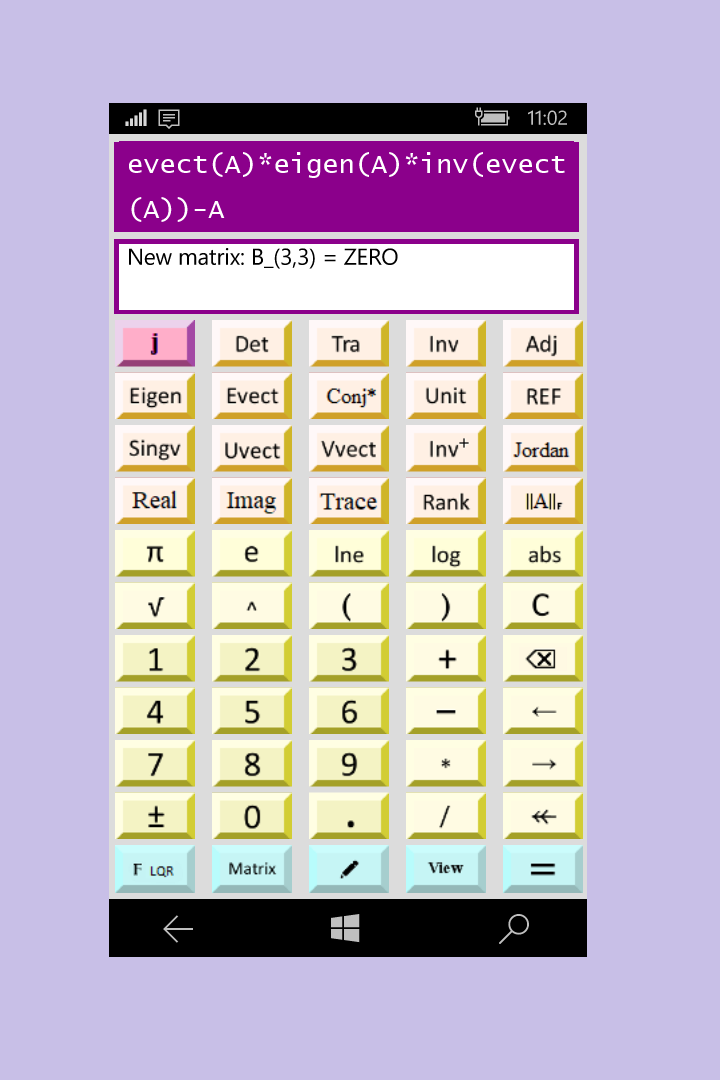¥13.00
¥13.00

## 介绍

The possible operations are the standard of mathematics and the following matrix operations: * = product matrix / = division of two matrices, or product of the inverse matrix ^ = power of a matrix (only with integers) + = sum matrix - = difference matrix Det = Determinant Tra = matrix transpose Inv = matrix inverse Adj = adjoint matrix tr(A) = trace of matrix A Unit = matrix unit Rank = matrix rank Erf = error function erf REF = matrix in Row Echelon Form (system solution) The following matrix operations are operative only with the Pro version: Inv+ = Moore - Penrose pseudo inverse Eigen = matrix eigenvalues Evect = matrix eigenvectors Vsing = matrix singular values S Uvect = left vector singular matrix U Vvect = right vector singular matrix V Dsum = matrix direct sum Outer = outer product L(L*L') = Lower triangolar matrix L so that A = L*L' Q(Q*R) = Left matrix Q so that A = Q*R R(Q*R) = Wright matrix R so thar A = Q*R Jordan = Jordan matrix J ||A|| = Frobenius norm e^A = exponential of matrix A √ A = square root matrix

## 其他信息

#### 发布者

Ing. Salvino Marras

#### 开发者

Ing. Salvino Marras

2019/5/2

21.89 MB

#### 支持的语言

English (United States)
Deutsch (Deutschland)
Español (España, Alfabetización Internacional)
Français (France)
Italiano (Italia)
Português (Portugal)
Русский (Россия)